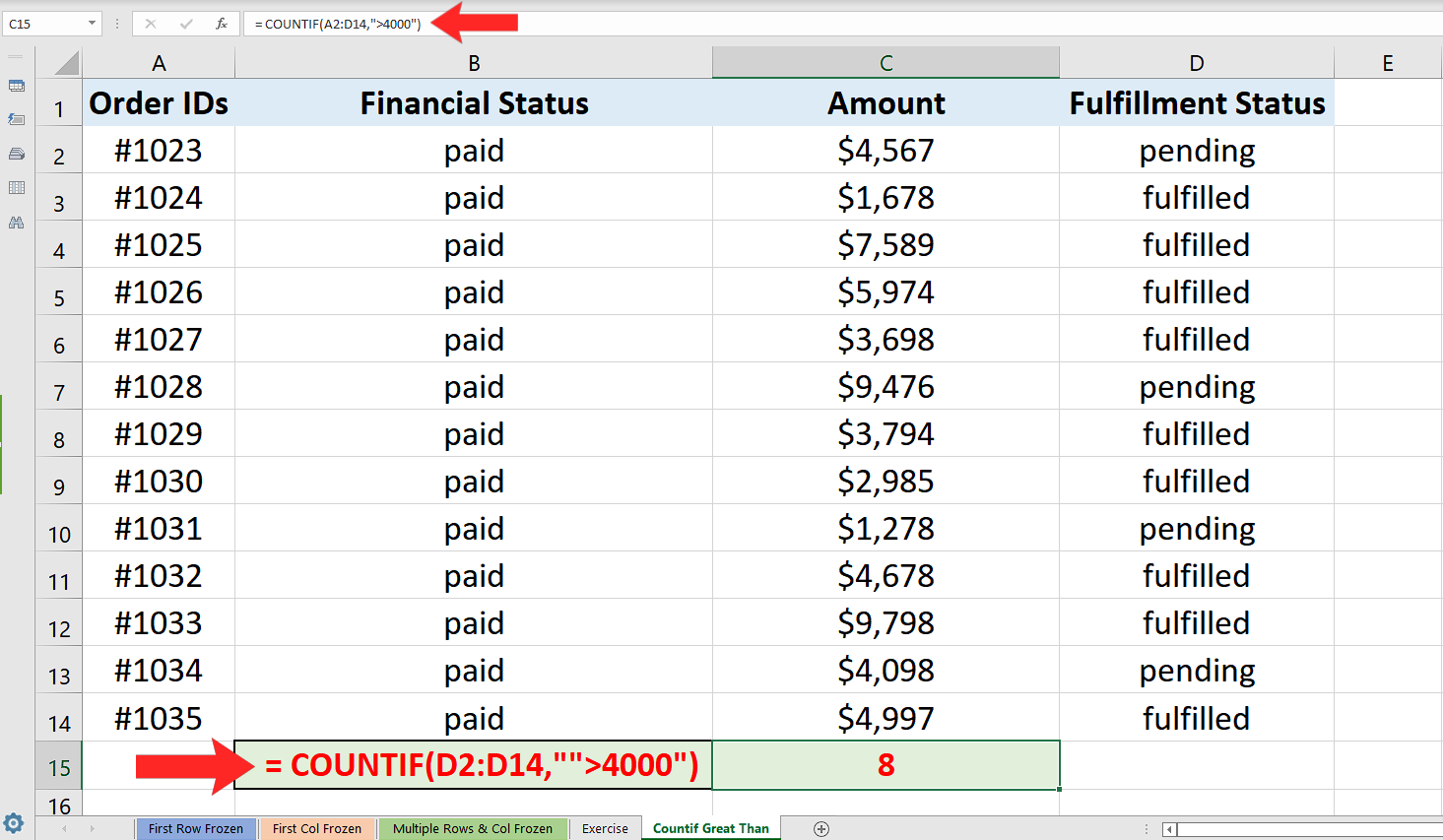# How to count cells Greater than a particular value in Excel

In this tutorial we will learn how we can use Excel’s built-in “COUNTIF” function to calculate the number of cells greater than a particular value. The best way to understand how countif works is to first understand the structure of the function and the parameters it requires.

## Structure of COUNTIF

The standard syntax of countif function in excel is shown below;

=COUNTIF(range, criteria)

• range (where to look in for something)

A range can be a single column with multiple rows or it could consist of multiple columns and multiple rows. For example “A1:D14”. This will tell the formula to search through two columns A & D till the 14th row.

• criteria (what to look for)

Criteria will be a simple logical test condition that will depend upon the current situation and the nature of values that we are looking for. In our current example we are looking for all those cells which are greater than a specific value so we will use a special logical condition in the formula which will tell Excel to count those cells which have values greater than a specific value.

Implementing the Formula on an Actual Data Set

take an example data set and find out the number of cells having values greater than a particular value only. So let’s consider the data set shown above.

In our data set we wish to find out the number of cells in which the paid amount is greater than 4000. In this case we can use the countif formula to find a solution to our problem. Let’s do this by following these steps;When analyzing real life data sets in Excel, we may come across a requirement to count those cells which have values greater than a particular value. Excel provides a very easy way to find out the number of cells greater than a particular value within a specific range.

### Step 1 – Implement the formula in a suitable cell– Choose a suitable cell where you wish to implement the formula. In this case we have chosen C15 to implement this formula.

– Use the following formula in that cell and press enter key.

= COUNTIF(C2:C14,”>4000″)

– The desired result will be displayed in this cell as soon as you will press the enter key as shown in the picture above.

That’s how it is done. We used COUNTIF with a special condition to count all rows in column C which have amounts greater than 4000 and successfully found out that there are 8 cells in which amounts are exceeding 4000 in the desired range.

## Breakdown of the Logical Condition used inside the formula

We used the formula COUNTIF(C2:C14,”>4000″). Let’s see how we told Excel where to look and what to look for.

• The first parameter C2:C14 tells Excel that the data range in which we are going to look for something is in column C and from row 2 to row 14 only.
• The second parameter was “>4000”. This condition tells Excel to look for all those cells that have values greater than 4000. When Excel finds a cell meeting this condition it increases the value of the counter. Our data set had eight cells which had amounts greater than 4000 in that range so the result was 8 as we desired.